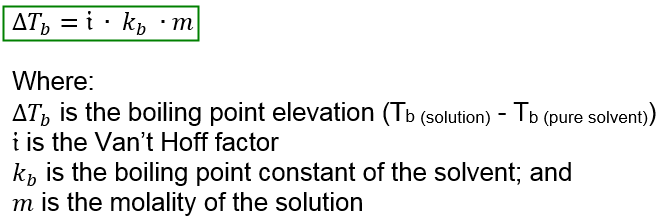# Problem: A solution contains 3.75 g of a nonvolatile pure hydrocarbon in 95 g acetone. The boiling points of pure acetone and the solution are 55.95°C and 56.50°C, respectively. The molal boiling point constant of acetone is 1.71 °C • kg/mol. What is the molar mass of the hydrocarbon?

###### FREE Expert Solution

Boiling point elevation is a colligative property that describes the increase of the boiling point of a solution when a solute is added to a pure solvent. The mathematical equation for boiling point elevation is:To solve for the molar mass of the hydrocarbon, we will:

1. Solve for the molality of the solution
2. Determine the mass in kg of the solvent
3. Calculate the molar mass of the hydrocarbon

84% (38 ratings)###### Problem Details

A solution contains 3.75 g of a nonvolatile pure hydrocarbon in 95 g acetone. The boiling points of pure acetone and the solution are 55.95°C and 56.50°C, respectively. The molal boiling point constant of acetone is 1.71 °C • kg/mol. What is the molar mass of the hydrocarbon?